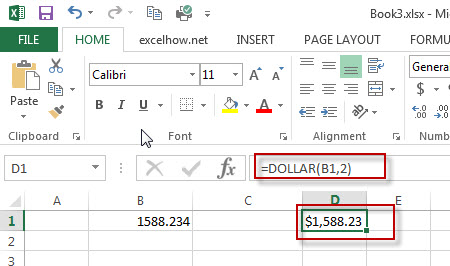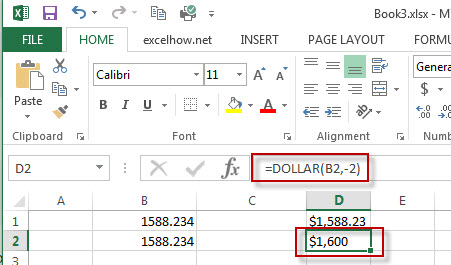# Excel Dollar Function

This post will guide you how to use Excel DOLLAR function with syntax and examples in Microsoft excel.

### Description

The Excel DOLLAR function converts a number to text using currency format (such as: \$ symbol), the currency format used depends upon your default language setting on your computer.

The DOLLAR function is a build-in function in Microsoft Excel and it is categorized as a Text Function.

The DOLLAR function is available in Excel 2016, Excel 2013, Excel 2010, Excel 2007, Excel 2003, Excel XP, Excel 2000, Excel 2011 for Mac.

### Syntax

The syntax of the DOLLAR function is as below:

= DOLLAR  (number, [decimals])

Where the DOLLAR function arguments are:
number -This is a required argument. The number to be converted into text string. Or a reference to a cell that containing a number.
Decimals-This is an optional argument. It will specify the number of decimal places to be displayed after decimal point.
Note: If Decimals value is omitted, it is set to be 2 by default.  If Decimals value is negative, the number is rounded to the left of the decimal point.

### Example

The below examples will show you how to use Excel DOLLAR Text function to convert a number as text using currency format.

#1 To convert Number value in B1 cell to a text string of a currency format and keep 2 digits to the right of the decimal point, just using DOLLAR(B1,2) formulas.#2 To Display the number in B2 in a currency format and keep 2 digits to the left of the decimal point, using excel formula: DOLLAR(B2,-2)